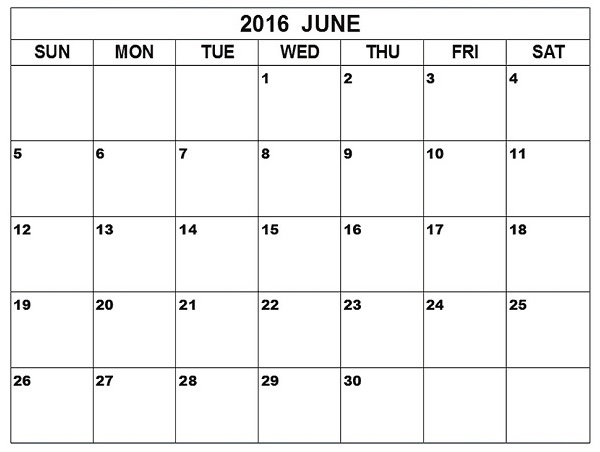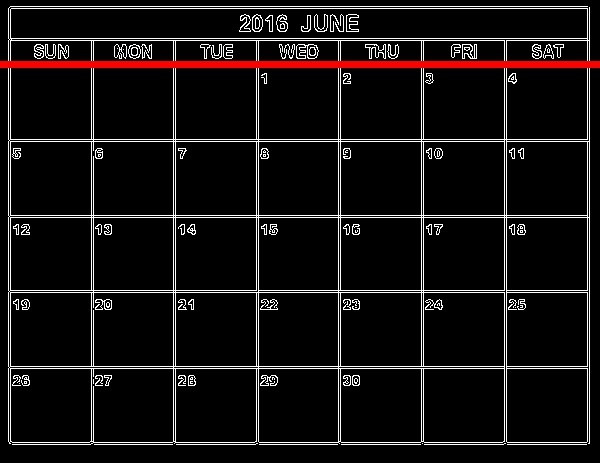# OpenCV - Hough Line Transform

You can detect the shape of a given image by applying the Hough Transform technique using the method HoughLines() of the Imgproc class. Following is the syntax of this method.

```HoughLines(image, lines, rho, theta, threshold)
```

This method accepts the following parameters −

• image − An object of the class Mat representing the source (input) image.

• lines − An object of the class Mat that stores the vector that stores the parameters (r, Φ) of the lines.

• rho − A variable of the type double representing the resolution of the parameter r in pixels.

• theta − A variable of the type double representing the resolution of the parameter Φ in radians.

• threshold − A variable of the type integer representing the minimum number of intersections to “detect” a line.

## Example

The following program demonstrates how to detect Hough lines in a given image.

```import org.opencv.core.Core;
import org.opencv.core.Mat;
import org.opencv.core.Point;
import org.opencv.core.Scalar;

import org.opencv.imgcodecs.Imgcodecs;
import org.opencv.imgproc.Imgproc;

public class HoughlinesTest {
public static void main(String args[]) throws Exception {

// Reading the Image from the file and storing it in to a Matrix object
String file = "E:/OpenCV/chap21/hough_input.jpg";

// Detecting edges of it
Mat canny = new Mat();
Imgproc.Canny(src, canny, 50, 200, 3, false);

// Changing the color of the canny
Mat cannyColor = new Mat();
Imgproc.cvtColor(canny, cannyColor, Imgproc.COLOR_GRAY2BGR);

// Detecting the hough lines from (canny)
Mat lines = new Mat();
Imgproc.HoughLines(canny, lines, 1, Math.PI/180, 100);

System.out.println(lines.rows());
System.out.println(lines.cols());

// Drawing lines on the image
double[] data;
double rho, theta;
Point pt1 = new Point();
Point pt2 = new Point();
double a, b;
double x0, y0;

for (int i = 0; i < lines.cols(); i++) {
data = lines.get(0, i);
rho = data;
theta = data;

a = Math.cos(theta);
b = Math.sin(theta);
x0 = a*rho;
y0 = b*rho;

pt1.x = Math.round(x0 + 1000*(-b));
pt1.y = Math.round(y0 + 1000*(a));
pt2.x = Math.round(x0 - 1000*(-b));
pt2.y = Math.round(y0 - 1000 *(a));
Imgproc.line(cannyColor, pt1, pt2, new Scalar(0, 0, 255), 6);
}
// Writing the image
Imgcodecs.imwrite("E:/OpenCV/chap21/hough_output.jpg", cannyColor);

System.out.println("Image Processed");
}
}
```

Assume that following is the input image hough_input.jpg specified in the above program.## Output

On executing the program, you will get the following output −

```143
1
Image Processed
```

If you open the specified path, you can observe the output image as follows −